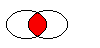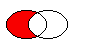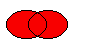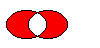# QRegion Class

The QRegion class specifies a clip region for a painter. More...

 Header: #include CMake: find_package(Qt6 REQUIRED COMPONENTS Gui) target_link_libraries(mytarget PRIVATE Qt6::Gui) qmake: QT += gui

## Public Types

 enum RegionType { Rectangle, Ellipse } const_iterator const_reverse_iterator

## Public Functions

 QRegion() QRegion(int x, int y, int w, int h, QRegion::RegionType t = Rectangle) QRegion(const QRect &r, QRegion::RegionType t = Rectangle) QRegion(const QPolygon &a, Qt::FillRule fillRule = Qt::OddEvenFill) QRegion(const QBitmap &bm) QRegion(const QRegion &r) QRegion(QRegion &&other) QRegion::const_iterator begin() const QRect boundingRect() const QRegion::const_iterator cbegin() const QRegion::const_iterator cend() const bool contains(const QPoint &p) const bool contains(const QRect &r) const QRegion::const_reverse_iterator crbegin() const QRegion::const_reverse_iterator crend() const QRegion::const_iterator end() const QRegion intersected(const QRegion &r) const QRegion intersected(const QRect &rect) const bool intersects(const QRegion ®ion) const bool intersects(const QRect &rect) const bool isEmpty() const bool isNull() const QRegion::const_reverse_iterator rbegin() const int rectCount() const QRegion::const_reverse_iterator rend() const void setRects(const QRect *rects, int number) QRegion subtracted(const QRegion &r) const void swap(QRegion &other) HRGN toHRGN() const void translate(int dx, int dy) void translate(const QPoint &point) QRegion translated(int dx, int dy) const QRegion translated(const QPoint &p) const QRegion united(const QRegion &r) const QRegion united(const QRect &rect) const QRegion xored(const QRegion &r) const QVariant operator QVariant() const bool operator!=(const QRegion &other) const QRegion operator&(const QRegion &r) const QRegion operator&(const QRect &r) const QRegion & operator&=(const QRegion &r) QRegion & operator&=(const QRect &r) QRegion operator+(const QRegion &r) const QRegion operator+(const QRect &r) const QRegion & operator+=(const QRegion &r) QRegion & operator+=(const QRect &rect) QRegion operator-(const QRegion &r) const QRegion & operator-=(const QRegion &r) QRegion & operator=(const QRegion &r) QRegion & operator=(QRegion &&other) bool operator==(const QRegion &r) const QRegion operator^(const QRegion &r) const QRegion & operator^=(const QRegion &r) QRegion operator|(const QRegion &r) const QRegion & operator|=(const QRegion &r)

## Static Public Members

 QRegion fromHRGN(HRGN hrgn)
 QDataStream & operator<<(QDataStream &s, const QRegion &r) QDataStream & operator>>(QDataStream &s, QRegion &r)

## Detailed Description

QRegion is used with QPainter::setClipRegion() to limit the paint area to what needs to be painted. There is also a QWidget::repaint() function that takes a QRegion parameter. QRegion is the best tool for minimizing the amount of screen area to be updated by a repaint.

This class is not suitable for constructing shapes for rendering, especially as outlines. Use QPainterPath to create paths and shapes for use with QPainter.

QRegion is an implicitly shared class.

### Creating and Using Regions

A region can be created from a rectangle, an ellipse, a polygon or a bitmap. Complex regions may be created by combining simple regions using united(), intersected(), subtracted(), or xored() (exclusive or). You can move a region using translate().

You can test whether a region isEmpty() or if it contains() a QPoint or QRect. The bounding rectangle can be found with boundingRect().

Iteration over the region (with begin(), end(), or C++11 ranged-for loops) gives a decomposition of the region into rectangles.

Example of using complex regions:

```void MyWidget::paintEvent(QPaintEvent *)
{
QRegion r1(QRect(100, 100, 200, 80),    // r1: elliptic region
QRegion::Ellipse);
QRegion r2(QRect(100, 120, 90, 30));    // r2: rectangular region
QRegion r3 = r1.intersected(r2);        // r3: intersection

QPainter painter(this);
painter.setClipRegion(r3);
// ...                                  // paint clipped graphics
}```

## Member Type Documentation

### enum QRegion::RegionType

Specifies the shape of the region to be created.

ConstantValueDescription
`QRegion::Rectangle``0`the region covers the entire rectangle.
`QRegion::Ellipse``1`the region is an ellipse inside the rectangle.

### QRegion::const_iterator

An iterator over the non-overlapping rectangles that make up the region.

The union of all the rectangles is equal to the original region.

QRegion does not offer mutable iterators.

### QRegion::const_reverse_iterator

A reverse iterator over the non-overlapping rectangles that make up the region.

The union of all the rectangles is equal to the original region.

QRegion does not offer mutable iterators.

## Member Function Documentation

### QRegion::QRegion()

Constructs an empty region.

### QRegion::QRegion(intx, inty, intw, inth, QRegion::RegionTypet = Rectangle)

Constructs a rectangular or elliptic region.

If t is `Rectangle`, the region is the filled rectangle (x, y, w, h). If t is `Ellipse`, the region is the filled ellipse with center at (x + w / 2, y + h / 2) and size (w ,h).

### QRegion::QRegion(const QRect &r, QRegion::RegionTypet = Rectangle)

Create a region based on the rectangle r with region type t.

If the rectangle is invalid a null region will be created.

### QRegion::QRegion(const QPolygon &a, Qt::FillRulefillRule = Qt::OddEvenFill)

Constructs a polygon region from the point array a with the fill rule specified by fillRule.

If fillRule is Qt::WindingFill, the polygon region is defined using the winding algorithm; if it is Qt::OddEvenFill, the odd-even fill algorithm is used.

Warning: This constructor can be used to create complex regions that will slow down painting when used.

### QRegion::QRegion(const QBitmap &bm)

Constructs a region from the bitmap bm.

The resulting region consists of the pixels in bitmap bm that are Qt::color1, as if each pixel was a 1 by 1 rectangle.

This constructor may create complex regions that will slow down painting when used. Note that drawing masked pixmaps can be done much faster using QPixmap::setMask().

### QRegion::QRegion(const QRegion &r)

Constructs a new region which is equal to region r.

### QRegion::QRegion(QRegion &&other)

Move-constructs a new region from region other. After the call, other is null.

### QRegion::const_iterator QRegion::begin() const

Returns a const_iterator pointing to the beginning of the range of non-overlapping rectangles that make up the region.

The union of all the rectangles is equal to the original region.

### QRect QRegion::boundingRect() const

Returns the bounding rectangle of this region. An empty region gives a rectangle that is QRect::isNull().

Same as begin().

Same as end().

### bool QRegion::contains(const QPoint &p) const

Returns `true` if the region contains the point p; otherwise returns `false`.

### bool QRegion::contains(const QRect &r) const

Returns `true` if the region overlaps the rectangle r; otherwise returns `false`.

### QRegion::const_reverse_iterator QRegion::crbegin() const

Same as rbegin().

Same as rend().

### QRegion::const_iterator QRegion::end() const

Returns a const_iterator pointing to one past the end of non-overlapping rectangles that make up the region.

The union of all the rectangles is equal to the original region.

### `[static, since 6.0] `QRegion QRegion::fromHRGN(HRGNhrgn)

Returns a QRegion that is equivalent to the given hrgn.

This function was introduced in Qt 6.0.

### QRegion QRegion::intersected(const QRegion &r) const

Returns a region which is the intersection of this region and r.The figure shows the intersection of two elliptical regions.

### QRegion QRegion::intersected(const QRect &rect) const

Returns a region which is the intersection of this region and the given rect.

### bool QRegion::intersects(const QRegion &region) const

Returns `true` if this region intersects with region, otherwise returns `false`.

### bool QRegion::intersects(const QRect &rect) const

Returns `true` if this region intersects with rect, otherwise returns `false`.

### bool QRegion::isEmpty() const

Returns `true` if the region is empty; otherwise returns `false`. An empty region is a region that contains no points.

Example:

```QRegion r1(10, 10, 20, 20);
r1.isEmpty();               // false

QRegion r3;
r3.isEmpty();               // true

QRegion r2(40, 40, 20, 20);
r3 = r1.intersected(r2);    // r3: intersection of r1 and r2
r3.isEmpty();               // true

r3 = r1.united(r2);         // r3: union of r1 and r2
r3.isEmpty();               // false```

### bool QRegion::isNull() const

Returns `true` if the region is empty; otherwise returns `false`. An empty region is a region that contains no points. This function is the same as isEmpty

### QRegion::const_reverse_iterator QRegion::rbegin() const

Returns a const_reverse_iterator pointing to the beginning of the range of non-overlapping rectangles that make up the region.

The union of all the rectangles is equal to the original region.

### int QRegion::rectCount() const

Returns the number of rectangles that this region is composed of. Same as `end() - begin()`.

### QRegion::const_reverse_iterator QRegion::rend() const

Returns a const_reverse_iterator pointing to one past the end of the range of non-overlapping rectangles that make up the region.

The union of all the rectangles is equal to the original region.

### void QRegion::setRects(const QRect *rects, intnumber)

Sets the region using the array of rectangles specified by rects and number. The rectangles must be optimally Y-X sorted and follow these restrictions:

• The rectangles must not intersect.
• All rectangles with a given top coordinate must have the same height.
• No two rectangles may abut horizontally (they should be combined into a single wider rectangle in that case).
• The rectangles must be sorted in ascending order, with Y as the major sort key and X as the minor sort key.

### QRegion QRegion::subtracted(const QRegion &r) const

Returns a region which is r subtracted from this region.The figure shows the result when the ellipse on the right is subtracted from the ellipse on the left (`left - right`).

### void QRegion::swap(QRegion &other)

Swaps region other with this region. This operation is very fast and never fails.

### `[since 6.0] `HRGN QRegion::toHRGN() const

Returns a HRGN that is equivalent to the given region.

This function was introduced in Qt 6.0.

### void QRegion::translate(intdx, intdy)

Translates (moves) the region dx along the X axis and dy along the Y axis.

### void QRegion::translate(const QPoint &point)

Translates the region point.x() along the x axis and point.y() along the y axis, relative to the current position. Positive values move the region to the right and down.

Translates to the given point.

### QRegion QRegion::translated(intdx, intdy) const

Returns a copy of the region that is translated dx along the x axis and dy along the y axis, relative to the current position. Positive values move the region to the right and down.

### QRegion QRegion::translated(const QPoint &p) const

Returns a copy of the regtion that is translated p.x() along the x axis and p.y() along the y axis, relative to the current position. Positive values move the rectangle to the right and down.

### QRegion QRegion::united(const QRegion &r) const

Returns a region which is the union of this region and r.The figure shows the union of two elliptical regions.

### QRegion QRegion::united(const QRect &rect) const

Returns a region which is the union of this region and the given rect.

### QRegion QRegion::xored(const QRegion &r) const

Returns a region which is the exclusive or (XOR) of this region and r.The figure shows the exclusive or of two elliptical regions.

### QVariant QRegion::operator QVariant() const

Returns the region as a QVariant

### bool QRegion::operator!=(const QRegion &other) const

Returns `true` if this region is different from the other region; otherwise returns `false`.

### QRegion QRegion::operator&(const QRegion &r) const

Applies the intersected() function to this region and r. `r1&r2` is equivalent to `r1.intersected(r2)`.

### QRegion &QRegion::operator&=(const QRegion &r)

Applies the intersected() function to this region and r and assigns the result to this region. `r1&=r2` is equivalent to `r1` = r1.intersected(r2).

### QRegion QRegion::operator+(const QRegion &r) const

Applies the united() function to this region and r. `r1+r2` is equivalent to `r1.united(r2)`.

### QRegion &QRegion::operator+=(const QRegion &r)

Applies the united() function to this region and r and assigns the result to this region. `r1+=r2` is equivalent to `r1 = r1.united(r2)`.

### QRegion &QRegion::operator+=(const QRect &rect)

Returns a region that is the union of this region with the specified rect.

### QRegion QRegion::operator-(const QRegion &r) const

Applies the subtracted() function to this region and r. `r1-r2` is equivalent to `r1.subtracted(r2)`.

### QRegion &QRegion::operator-=(const QRegion &r)

Applies the subtracted() function to this region and r and assigns the result to this region. `r1-=r2` is equivalent to `r1 = r1.subtracted(r2)`.

### QRegion &QRegion::operator=(const QRegion &r)

Assigns r to this region and returns a reference to the region.

### QRegion &QRegion::operator=(QRegion &&other)

Move-assigns other to this QRegion instance.

### bool QRegion::operator==(const QRegion &r) const

Returns `true` if the region is equal to r; otherwise returns false.

### QRegion QRegion::operator^(const QRegion &r) const

Applies the xored() function to this region and r. `r1^r2` is equivalent to `r1.xored(r2)`.

### QRegion &QRegion::operator^=(const QRegion &r)

Applies the xored() function to this region and r and assigns the result to this region. `r1^=r2` is equivalent to `r1 = r1.xored(r2)`.

### QRegion QRegion::operator|(const QRegion &r) const

Applies the united() function to this region and r. `r1|r2` is equivalent to `r1.united(r2)`.

### QRegion &QRegion::operator|=(const QRegion &r)

Applies the united() function to this region and r and assigns the result to this region. `r1|=r2` is equivalent to `r1 = r1.united(r2)`.

## Related Non-Members

### QDataStream &operator<<(QDataStream &s, const QRegion &r)

Writes the region r to the stream s and returns a reference to the stream.